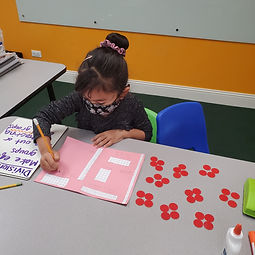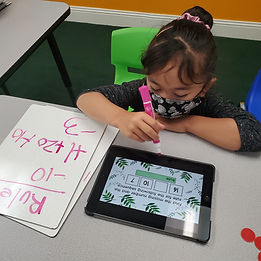## Ms. Raejean

### Target 1​

###### Lesson Type:

Continuation

Number Operation

:

Computation

Divide within 100.

###### 1:

Understand that dividing numbers makes numbers smaller.

###### 2:

Understand that division represents taking a quantity and determining how many equal groups of objects can be made.

###### 3:

Understand there are multiple ways to divide a multi-digit number.

###### 4:

Understand that division equations can be written both vertically and horizontally and division can be indicated using multiple symbols (i.e., ÷, ⟌).

3rd

###### Vocabulary:

Division, Divide, Multi-Digit

Activities:

Students continued working on division concepts. We focused on the idea that multi-digit numbers can be divided in multiple ways. Students used counters to divide 2-digit numbers in different ways - making groups of 2, 3, 4, etc. (ex. 12/2 = 6, 12/3 = 4, etc.) Students also made arrays to represent the amount being divided in multiple ways and wrote the equations. (ex. 24/2 = 12, 24/4 = 6, 24/3 = 8, 24/1=24)### Home Exploration

###### Guiding Questions:## Absent Students:

### Target 2

:

###### 1:

Study a given arithmetic sequence to determine what rule explains the sequence (i.e., 2-4-6-8-10…each number is the sequence gets bigger by 2).

###### 2:

Write an arithmetic rule to explain a given sequence.

###### 3:

Understand that a pattern can be described by stating the rule that it is following.

3rd

###### Vocabulary:

Pattern, Sequence

Activities:

Students looked at an arithmetic sequence, determined the rule, and identified the missing number. If needed, students used counters to help find the difference between numbers to determine how the numbers in the sequence changed.### Home Exploration

###### Guiding Questions:### Target 3

:

###### Vocabulary:

Activities:### Home Exploration